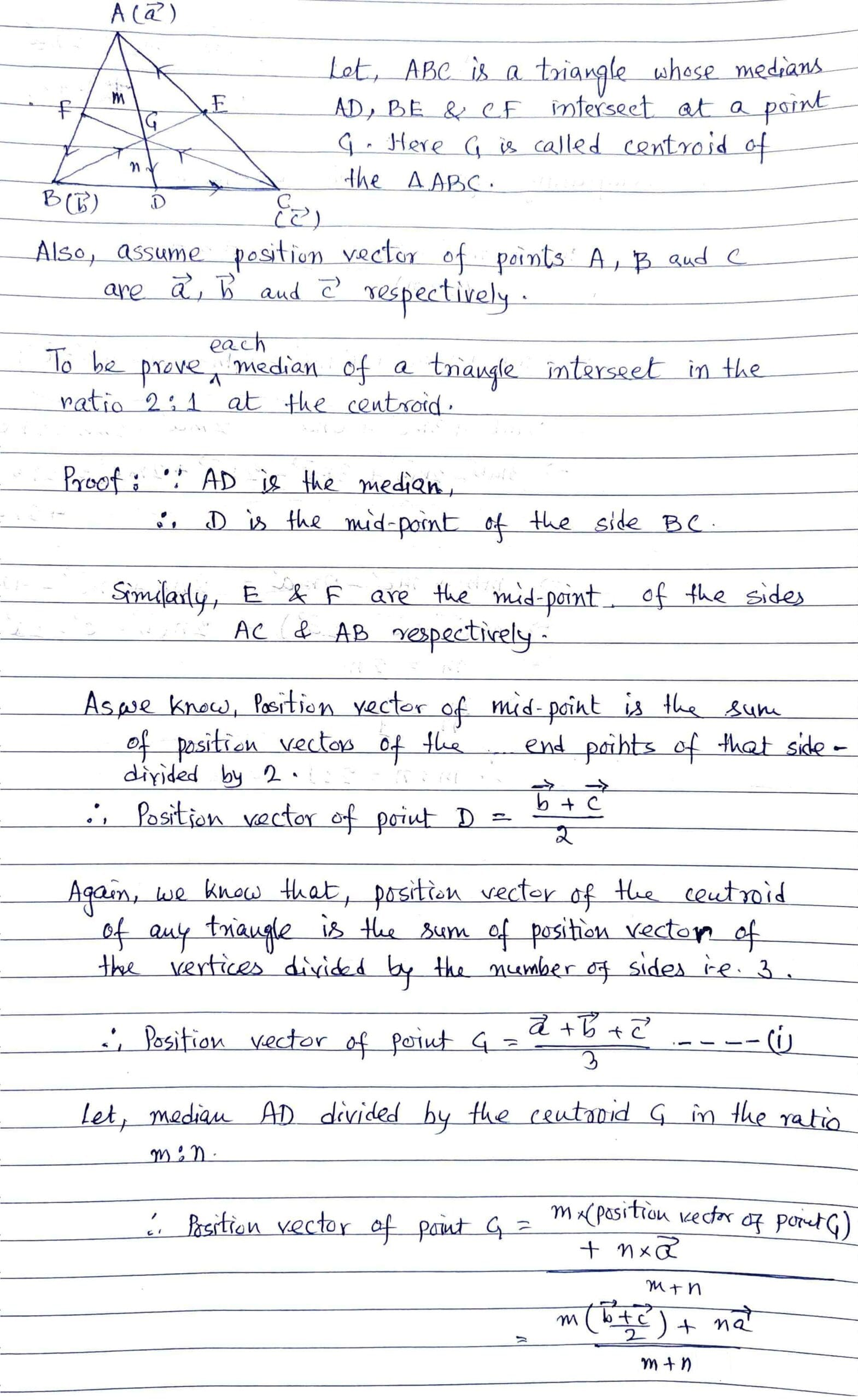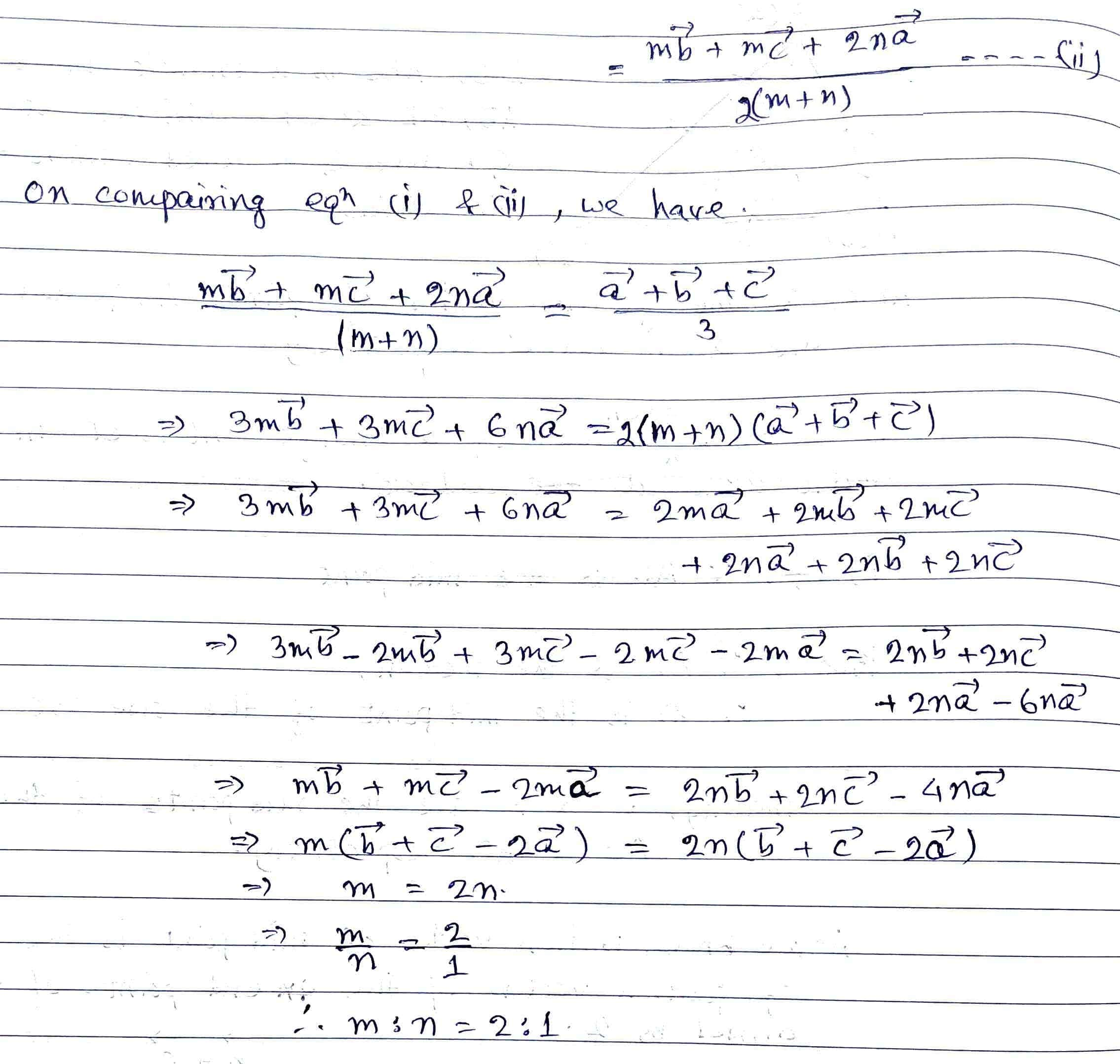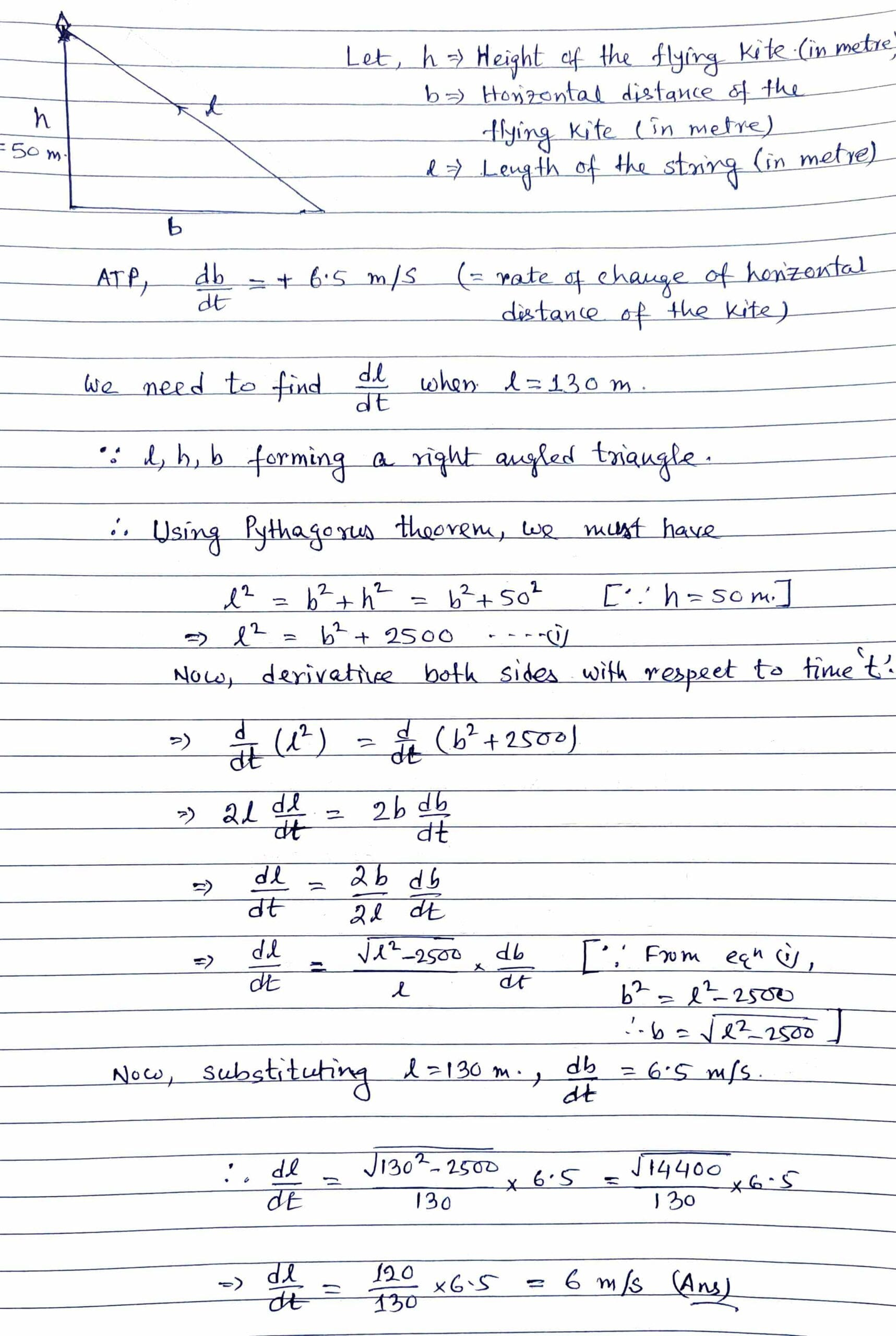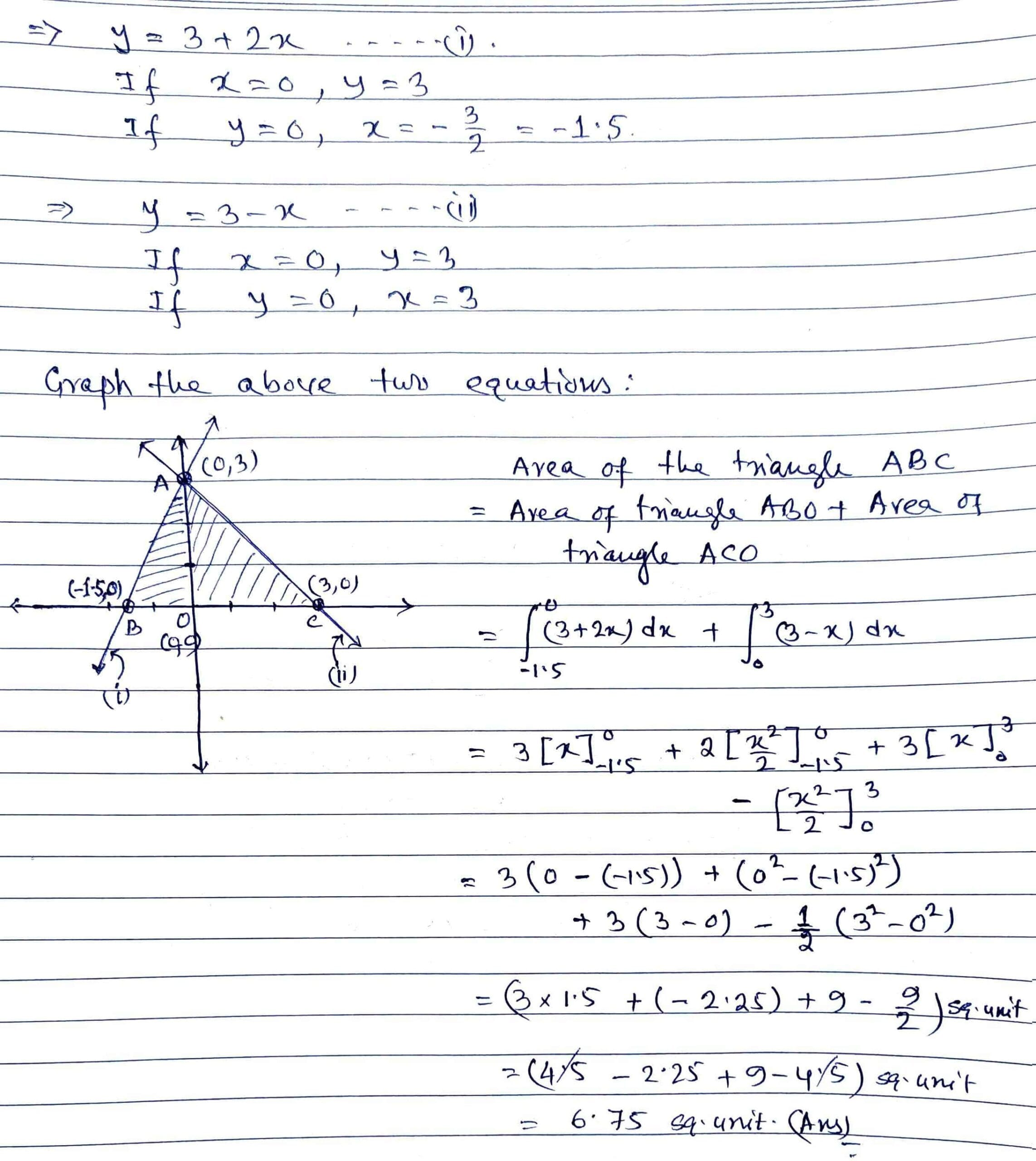Tue. Sep 26th, 2023

Aug 30, 2022

# BCS-012 June 2022 Solution

Q1 (a): Solve the following system of linear equations using Cramer’s rule:

x + y = 0;  y + z = 1;  z + x = 3

Solution: Refer to BCS-12 June 2021 Part 5 [Q4 (c)]

Time Stamp : 13:57 (Must see the description)

Q1 (b): If  1, ω  and  ω2  are cube roots of unity, show that  (2 − ω)(2 − ω2)(2 − ω10)(2 − ω11) = 49.

Solution: Refer to BCS-12 December 2020 Part 4 [Q3 (b)]

Time Stamp : 13:21 (Must see the description)

Q1 (c): Evaluate the integral

Solution: Refer to BCS-12 June 2021 Part 2 [Q1 (h)]

Time Stamp : 49:03 (Must see the description)

Q1 (d): Solve the inequality

Solution: Refer to BCS-12 June 2021 Part 2 [Q1 (g)]

Time Stamp : 33:23 (Must see the description)

Q1 (e): Show that

Solution: Refer to BCS-12 December 2020 Part 1 [Q1 (a)]

Time Stamp : 00:16 (Must see the description)

Q1 (f): Find the quadratic equation whose roots are  (2 − √3)  and  (2 + √3).

Solution: Refer to BCS-12 December 2021 [Q1 (g)]

Q1 (g): Find the sum of an infinite G.P. whose first term is 28 and fourth term is  4/49.

Solution: Refer to BCS-12 December 2020 Part 4 [Q3 (d)]

Time Stamp : 29:34 (Must see the description)

Q1 (h): If  Z  is a complex number such that  |Z − 2i| = |Z + 2i|,  show that  Im (Z) = 0.

Solution: Refer to BCS-12 December 2021 [Q1 (c)]

Q2 (a): Evaluate

Solution: Refer to BCS-12 December 2020 Part 2 [Q1 (d)]

Time Stamp : 00:16 (Must see the description)

Q2 (b): Prove that the three medians of a triangle meet at a point called centroid of the triangle which divides each of the medians in the ratio of 2 : 1.

Solution:Q2 (c): A young child is flying kite which is at a height of 50 m. The wind is carrying the kite horizontally away from the child at a speed of 6.5 m/s. How fast must the kite string be let out when the string is 130 m ?

Solution:Q3 (a): Using Principle of Mathematical Induction, show that  n(n + 1)(2n + 1)  is a multiple of  6  for every natural number  n.

Solution:Q3 (b): Find the points of local minima and local maxima for

Solution: Refer to BCS-12 December 2021 [Q2 (b)]

Q3 (c): Determine the 100th term of the Harmonic progression

Solution: Refer to BCS-12 December 2021 [Q3 (c)]

Q3 (d): Find the length of the curve  y = 2x 3/2  from (1, 2) to (4, 16).

Solution: Refer to BCS-12 December 2020 Part 5 [Q4 (b)]

Time Stamp : 17:58 (Must see the description)

Q4 (a): Determine the shortest distance between the lines

and

Solution: Refer to BCS-12 December 2021 [Q2 (a)]

Q4 (b): Find the area lying between two curves

y = 3 + 2xy = 3 − x,  0 ≤ x ≤ 3

using integration.

Solution:Q4 (c): If   , prove that

Solution: Refer to BCS-12 June 2021 Part 2 [Q1 (e)]

Time Stamp : 07:30 (Must see the description)

Q4 (d): Find the angle between the lines

and

Solution: Refer to BCS-12 June 2021 Part 1 [Q1 (c)]

Time Stamp : 23:18 (Must see the description)

Q5 (a): If  , show that  A(adj A) = O.

Solution:Q5 (b): Use De Moivre’s theorem to find  (√3 + 1)3.

Solution: Refer to BCS-12 June 2021 Part 3 [Q2 (d)]

Time Stamp : 28:24 (Must see the description)

Q5 (c): Show that      is perpendicular to  ,  for any two non zero vectors    and   .

Solution: Refer to BCS-12 December 2021 [Q1 (d)]

Q5 (d): If  , find  .

Solution: Refer to BCS-12 June 2021 Part 5 [Q4 (d)]

Time Stamp : 25:48 (Must see the description)

#### Navigating JENPAUH 2021: Your Gateway to a Bright Medical Career

error: Content is protected !!